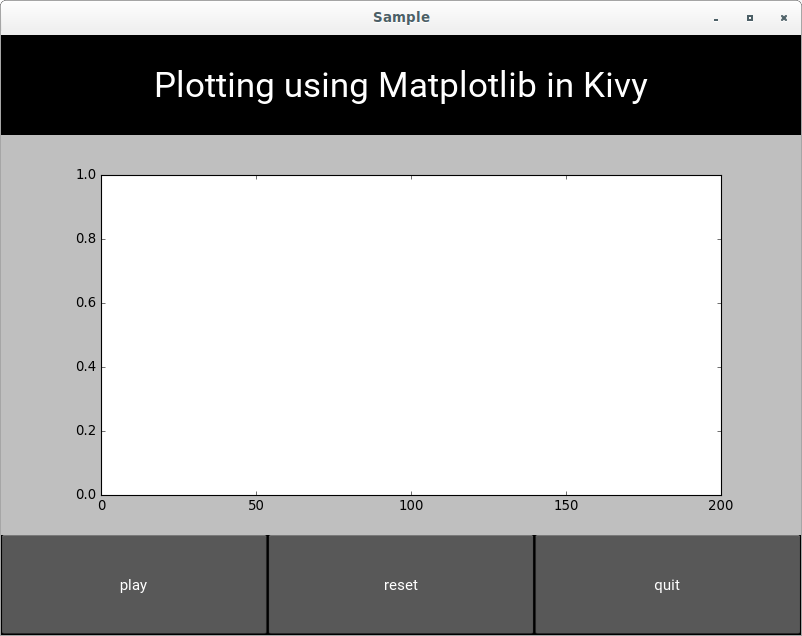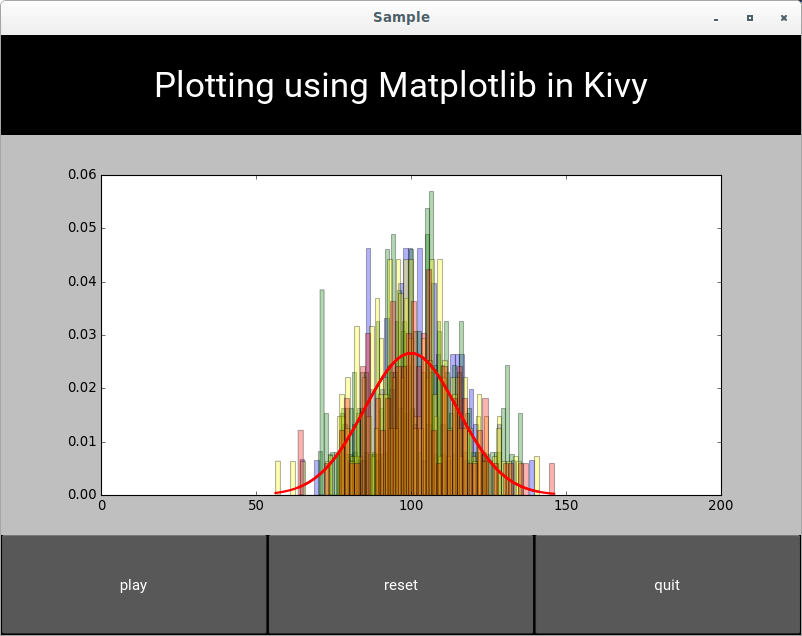## Matplotlib adding to existing plots

Date: March 5th 2016
Last updated: March 5th 2016

In this example, each click on the play button renders additional attributes to a figure. I didn't arrive at this intentionally. I was actually trying to replace each figure when the play button was clicked. I imagine that this code will come in handy at some point in the future.

main.py

``````"""
Keeps the same graph 'window'.
Plots new bins overtop of existing plot.
"""

import matplotlib
matplotlib.use('module://kivy.garden.matplotlib.backend_kivy')
import matplotlib.pyplot as plt
from kivy.garden.matplotlib.backend_kivyagg import FigureCanvas
import kivy
kivy.require('1.9.1')
from kivy.app import App
from kivy.uix.boxlayout import BoxLayout
from kivy.uix.button import Button
import matplotlib.mlab as mlab
from random import gauss
import random
import numpy as np

class DrawPlot(BoxLayout):
def __init__(self, **kwargs):
super(DrawPlot, self).__init__(**kwargs)

def get_data(self):
x = [gauss(100, 15) for i in range(100)]
return x

def draw_plot_frame(self):
self.fig, self.ax = plt.subplots()
self.ax.set_xlim([0, 200])
canvas =  FigureCanvas(figure=self.fig)
self.add_widget(canvas, 1) #<===== builds initial plot window

def plot(self):
x = self.get_data()
colorlist = ['blue','red','green','yellow']
facecolor = colorlist[random.randint(0,3)]
n, bins, patches = self.ax.hist(x, 50, normed=1, facecolor=facecolor, alpha=0.3)
pdf = mlab.normpdf(bins, 100, 15)
self.ax.plot(bins, pdf, 'r-', linewidth=2)
canvas = self.fig.canvas
canvas.draw() #<===== this adds stuff from plot() to the existing plot window

class SampleApp(App):
def build(self):
return DrawPlot()

if __name__ == '__main__':
SampleApp().run()
``````

sample.kv

``````#:kivy 1.9.1
<DrawPlot>:
orientation: 'vertical'

BoxLayout:
orientation: 'vertical'
size_hint: 1, None
height: main_title.height

Label:
id: main_title
text: "Plotting using Matplotlib in Kivy"
font_size: '35sp'
size_hint: 1, None
height: self.height

Label:
size_hint_x: 0.8
source: root.draw_plot_frame() #<==== creates initial plot window

BoxLayout:
orientation: 'horizontal'
size_hint: 1, None
height: playbutton.height
Button:
id: playbutton
text: 'play'
size_hint: 1, None
height: self.height
on_press: root.plot() #<==== add stuff to existing plot

Button:
text: 'reset'
size_hint: 1, None
Button:
text: 'quit'
size_hint: 1, None
``````

Output

The following screenshot is produced when running the main program.After clicking on the play button several times I got the following image. Note that the colours are chosen at random (blue, red, green, yellow) to fill in the histogram bins. Reset and quit buttons do nothing.